# Oxidation-Reduction Potential (ORP) - The Basis of its Measurement

Oxidation reduction potential (ORP), often known as redox potential, is the electric potential based on the equilibrium conditions between oxidants (Mz+) and reductants (M(z-n)+) in a solution.

Suppose, equilibrium (1) exists in a solution: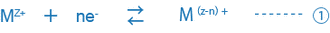If a metal electrode (e.g., platinum, gold) and reference electrode are placed in this solution, the potential difference between the electrodes, or ORP (mV) can be measured using a potentiometer, and the potential difference expressed as: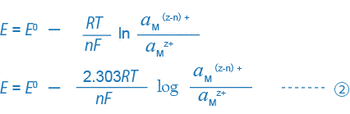When trivalent and bivalent iron ions coexist in a solution, equations (1) and (2) can be expressed as: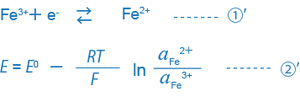When the only condition in the solution is equation (1)’, the ORP is given by (2)’. It is key to note that here, the ORP is given by the ratio of the activity of the reductant (Fe2+) to that of the oxidant (Fe3+). However, in reality, many different equilibrium conditions are present in a solution among different ions at any given time. Hence, the ORP of such a solution cannot be given by such a simple equation, and the physical interpretation of the ORP determined using this equation is not clear.

ORP of a solution is one measure of the properties of a solution. It is widely used in analyzing solutions or as an indicator in wastewater treatment. Recently, it has been suggested that a high ORP can sterilize water, and drinking water with low ORP can make one more immune to diseases as a result of the reaction with the active oxygen in the body cells. ORPs are also used as an indicator for alkaline drinking water.

## Types of Reference Electrodes and ORP (mV)

A measured ORP is the value for the reference electrode used, so it is depends on the type of reference electrode. HORIBA’s reference electrodes use Ag/AgCl with a 3.33 mol/L KCl internal solution.

Generally, standard hydrogen electrodes (S.H.E. or N.H.E.) are used as reference electrodes in academic papers. The ORP for N.H.E. as the reference electrode when Ag/AgCl is used as the reference electrode is given by: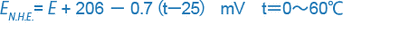EN.H.E. :ORP (mV) measurement when a normal hydrogen electrode (N.H.E.) is used as the reference electrode

E :ORP (mV) measurement when a Ag/AgCl electrode with 3.33 mol/L KCl is used as the reference electrode

### Positive and Negative Signs of Electric Potentials

Standard oxidation-reduction potentials are shown in a variety of chemistry related books as: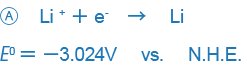However, in some books, particularly those written in the US, it is written in the opposite way, with positive and negative signs reversed:Although both express the same reaction, the way the signs are used may be confusing. The format used in A is the standard generally used around the world and is the way ORPs are also expressed.

This treaty is titled the Stockholm Convention of the International Union of Pure and Applied Chemistry (IUPAC). The signs are used so that the arrows indicate the direction in which the main chemical entities change from an oxidant to a reductant.This information has been sourced, reviewed and adapted from materials provided by HORIBA Scientific.

For more information on this source, please visit HORIBA Scientific.

## Citations

Please use one of the following formats to cite this article in your essay, paper or report:

• APA

HORIBA Scientific. (2019, February 12). Oxidation-Reduction Potential (ORP) - The Basis of its Measurement. AZoM. Retrieved on September 18, 2019 from https://www.azom.com/article.aspx?ArticleID=16139.

• MLA

HORIBA Scientific. "Oxidation-Reduction Potential (ORP) - The Basis of its Measurement". AZoM. 18 September 2019. <https://www.azom.com/article.aspx?ArticleID=16139>.

• Chicago

HORIBA Scientific. "Oxidation-Reduction Potential (ORP) - The Basis of its Measurement". AZoM. https://www.azom.com/article.aspx?ArticleID=16139. (accessed September 18, 2019).

• Harvard

HORIBA Scientific. 2019. Oxidation-Reduction Potential (ORP) - The Basis of its Measurement. AZoM, viewed 18 September 2019, https://www.azom.com/article.aspx?ArticleID=16139.

## Ask A Question

Do you have a question you'd like to ask regarding this article?